SLES023D December   2001  – December 2016

PRODUCTION DATA.

1. Features
2. Applications
3. Description
4. Revision History
5. Pin Configuration and Functions
6. Specifications
7. Detailed Description
1. 7.1 Overview
2. 7.2 Functional Block Diagrams
3. 7.3 Feature Description
4. 7.4 Device Functional Modes
8. Application and Implementation
1. 8.1 Application Information
2. 8.2 Typical Application
1. 8.2.1 Design Requirements
2. 8.2.2 Detailed Design Procedure
3. 8.2.3 Application Curve
9. Power Supply Recommendations
10. 10Layout
1. 10.1 Layout Guidelines
2. 10.2 Layout Example
11. 11Device and Documentation Support
12. 12Mechanical, Packaging, and Orderable Information

• DB|20

## 6 Specifications

### 6.1 Absolute Maximum Ratings

over operating free-air temperature range (unless otherwise noted)(1)
MIN MAX UNIT
Supply voltage VCC 6.5 V
VDD 4
Ground voltage differences AGND and DGND ±0.1 V
Supply voltage difference (VCC – VDD) VCC and VDD 3 V V
Digital input voltage FSYNC, LRCK, BCK, and DOUT –0.3 VDD + 0.3 V
PDWN, BYPAS, SCKI, OSR, FMT0, FMT1, MODE0, and MODE1 –0.3 6.5
Analog input voltage VINL, VINR, VREF1, and VREF2 –0.3 VCC + 0.3 V
Input current (any pins except supplies) ±10 mA
Ambient temperature under bias –40 125 °C
Junction temperature 150 °C
Package temperature (IR reflow, peak) 260 °C
Storage temperature, Tstg –55 150 °C
Stresses beyond those listed under Absolute Maximum Ratings may cause permanent damage to the device. These are stress ratings only, which do not imply functional operation of the device at these or any other conditions beyond those indicated under Recommended Operating Conditions. Exposure to absolute-maximum-rated conditions for extended periods may affect device reliability.

### 6.2 ESD Ratings

VALUE UNIT
V(ESD) Electrostatic discharge Human-body model (HBM), per ANSI/ESDA/JEDEC JS-001(1) ±1500 V
Charged-device model (CDM), per JEDEC specification JESD22-C101(2) ±1000
JEDEC document JEP155 states that 500-V HBM allows safe manufacturing with a standard ESD control process.
JEDEC document JEP157 states that 250-V CDM allows safe manufacturing with a standard ESD control process.

### 6.3 Recommended Operating Conditions

over operating free-air temperature range (unless otherwise noted)
MIN NOM MAX UNIT
Analog supply voltage, VCC 5 V
Digital supply voltage, VDD 3.3 V
Digital input logic family TTL
Digital input clock frequency Sampling clock 8.192 49.152 MHz
System clock 32 96 kHz
Digital output load capacitance 20 pF
Operating free-air temperature, TA –40 85 °C

### 6.4 Thermal Information

THERMAL METRIC(1) PCM1802 UNIT
DB (SSOP)
20 PINS
RθJA Junction-to-ambient thermal resistance 80.8 °C/W
RθJC(top) Junction-to-case (top) thermal resistance 40 °C/W
RθJB Junction-to-board thermal resistance 37.6 °C/W
ψJT Junction-to-top characterization parameter 7.2 °C/W
ψJB Junction-to-board characterization parameter 37 °C/W

### 6.5 Electrical Characteristics

TA = 25°C, VCC = 5 V, VDD = 3.3 V, master mode, fS = 44.1 kHz, system clock = 384 fS, oversampling ratio = ×128, 24-bit data (unless otherwise noted)
PARAMETER TEST CONDITIONS MIN TYP MAX UNIT
Resolution 24 Bits
DATA FORMAT
Audio data interface format Left-justified, I2S, or rightjustified
Audio data bit length 20 or 24 Bits
Audio data format MSB first or 2s complement
fS Sampling frequency 16 44.1 96 kHz
System clock frequency 256 fS 4.096 11.2896 24.576 MHz
384 fS 6.144 16.9344 36.864
512 fS 8.192 22.5792 49.152
768 fS(1) 12.288 33.8688
INPUT LOGIC
VIH Input logic level(2) 2 VDD VDC
VIL 0 0.8
VIH Input logic level(3) 2 5.5
VIL 0 0.8
IIH Input logic current(4) VIN = VDD ±10 µA
IIL VIN = 0 V ±10
IIH Input logic current(5) VIN = VDD 65 100
IIL VIN = 0 V ±10
OUTPUT LOGIC
VOH Output logic level(6) IOUT = –1 mA 2.8 VDC
VOL IOUT = 1 mA 0.5
DC ACCURACY
Gain mismatch,
channel-to-channel
±1% ±4% FSR
Gain error ±2% ±6% FSR
Bipolar zero error HPF bypassed(7) ±2% FSR
DYNAMIC PERFORMANCE(8)
THD+N Total harmonic distortion + noise fS = 44.1 kHz, VIN = –0.5 dB 0.0015% 0.003%
fS = 96 kHz, VIN = –0.5 dB, system clock = 256 fS, oversampling ratio = ×64(9) 0.0025%
fS = 44.1 kHz, VIN = –60 dB 0.7%
fS = 96 kHz, VIN = –60 dB, system clock = 256 fS, oversampling ratio = ×64(9) 1.2%
Dynamic range fS = 44.1 kHz, A-weighted 100 105 dB
fS = 96 kHz, A-weighted, system clock = 256 fS, oversampling ratio = ×64(9) 103
Signal to noise ratio fS = 44.1 kHz, A-weighted 100 105 dB
fS = 96 kHz, A-weighted, system clock = 256 fS, oversampling ratio = ×64(9) 103
Channel separation fS = 44.1 kHz 96 103 dB
fS = 96 kHz, system clock = 256 fS, oversampling ratio = ×64(9) 98
Input voltage 0.6 × VCC VP–P
VREF1 Center voltage 0.5 × VCC V
Input impedance 20
Antialiasing filter frequency response –3 dB 300 kHz
DIGITAL FILTER PERFORMANCE
Pass band 0.454 fS Hz
Stop band 0.583 fS Hz
Pass-band ripple ±0.05 dB
Stop-band attenuation –65 dB
Delay time 17.4 / fS s
HPF frequency response –3 dB 0.019 fS mHz
POWER SUPPLY REQUIREMENTS
VCC Voltage 4.5 5 5.5 VDC
VDD 2.7 3.3 3.6
ICC Supply current(10) VCC = 5 V, VDD = 3.3 V 24 30 mA
IDD fS = 44.1 kHz VCC = 5 V, VDD = 3.3 V 8.3 10
fS = 96 kHz, VCC = 5 V, VDD = 3.3 V(8) 17
PD Power dissipation Operation fS = 44.1 kHz, VCC = 5 V, VDD = 3.3 V 147 183 mW
fS = 96 kHz, VCC = 5 V, VDD = 3.3 V(8) 176
Power down VCC = 5 V, VDD = 3.3 V 0.5
Maximum system clock frequency is not applicable at 768 fS, fS = 96 kHz (see System Clock).
Applies to FSYNC, LRCK, BCK (Schmitt-trigger input in slave mode) pins.
Applies to PDWN, BYPAS, SCKI, OSR, FMT0, FMT1, MODE0, MODE1 (Schmitt-trigger input, 5-V tolerant) pins.
Applies to FSYNC, LRCK, BCK (Schmitt-trigger input in slave mode), SCKI (Schmitt-trigger input) pins.
Applies to PDWN, BYPAS, OSR, FMT0, FMT1, MODE0, MODE1 (Schmitt-trigger input, with 50-kΩ typical pulldown resistor) pins.
Applies to FSYNC, LRCK, BCK (in master mode), DOUT pins.
High-pass filter
Analog performance specifications are tested with System Two™ audio measurement system by Audio Precision™, using 400-Hz HPF, 20-kHz LPF for 44.1-kHz operation or 40-kHz LPF for 96-kHz operation in RMS mode.
fS = 96 kHz, system clock = 256 fS, oversampling ratio = ×64.
Minimum load on DOUT, BCK, LRCK, and FSYNC.

### 6.6 Typical Characteristics

TA = 25°C, VCC = 5 V, VDD = 3.3 V, Master mode, fS = 44.1 kHz, system clock = 384 fS, oversampling ratio = ×128, and 24-bit data (unless otherwise noted).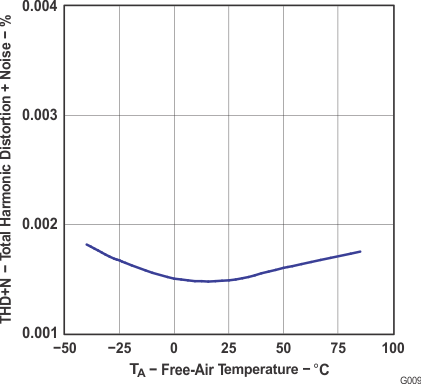Figure 1. Total Harmonic Distortion + Noise
vs Free-Air Temperature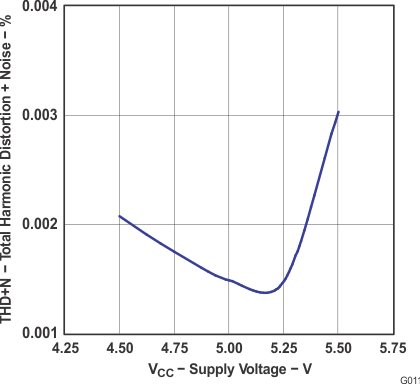Figure 3. Total Harmonic Distortion + Noise
vs Supply VoltageFigure 5. Total Harmonic Distortion + Noise
vs fSAMPLE Condition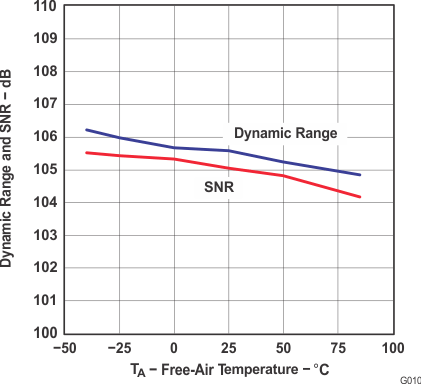Figure 2. Dynamic Range and SNR
vs Free-Air Temperature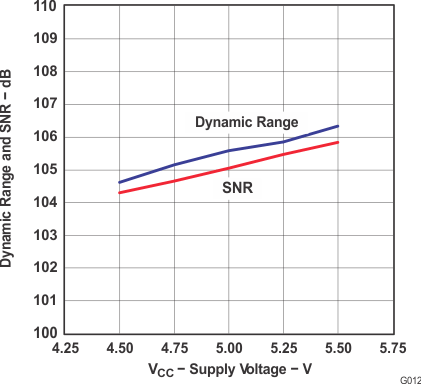Figure 4. Dynamic Range and SNR vs Suppy Voltage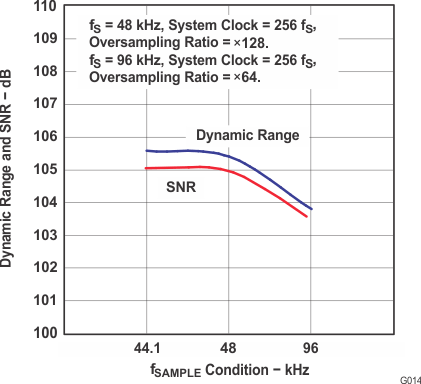Figure 6. Dynamic Range and SNR vs fSAMPLE Condition

#### 6.6.1.1 Digital Filter: Decimation Filter Frequency Response

TA = 25°C, VCC = 5 V, VDD = 3.3 V, Master mode, fS = 44.1 kHz, system clock = 384 fS, oversampling ratio = ×128, and 24-bit data (unless otherwise noted).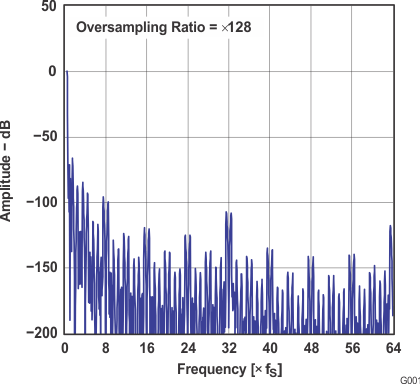Figure 7. Amplitude vs Frequency Overall Characteristics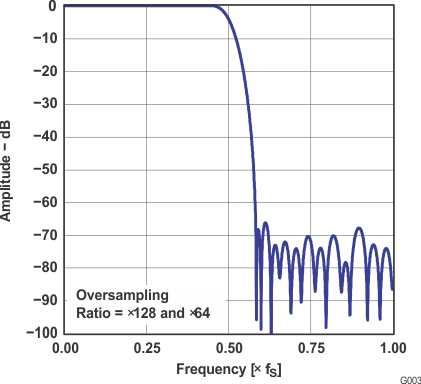Figure 9. Amplitude vs Frequency Stop-Band Attenuation Characteristics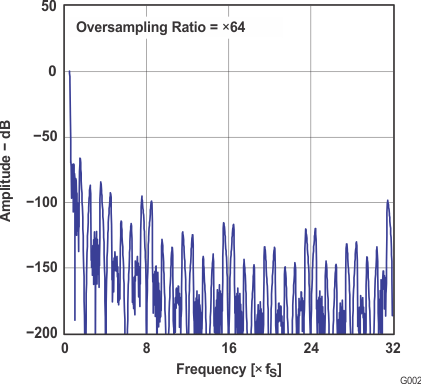Figure 8. Amplitude vs Frequency Overall Characteristics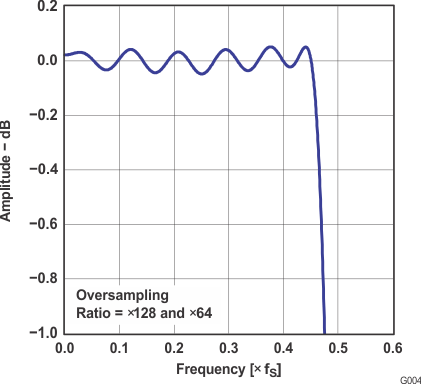Figure 10. Amplitude vs Frequency Pass-Band Ripple Characteristics

#### 6.6.1.2 HPF (High-Pass Filter) Frequency Response

TA = 25°C, VCC = 5 V, VDD = 3.3 V, Master mode, fS = 44.1 kHz, system clock = 384 fS, oversampling ratio = ×128, and 24-bit data (unless otherwise noted).Figure 11. Amplitude vs Frequency HPF
Stop-Band Characteristics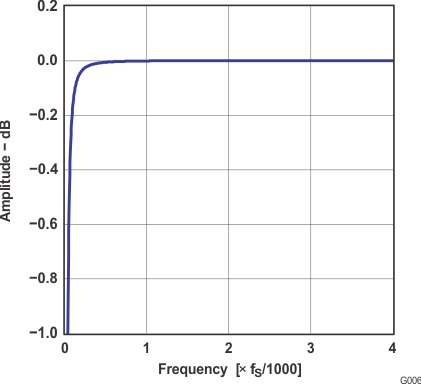Figure 12. Amplitude vs Frequency HPF
Pass-Band Characteristics

#### 6.6.1.3 Analog Filter: Antialiasing Filter Frequence Response

TA = 25°C, VCC = 5 V, VDD = 3.3 V, Master mode, fS = 44.1 kHz, system clock = 384 fS, oversampling ratio = ×128, and 24-bit data (unless otherwise noted).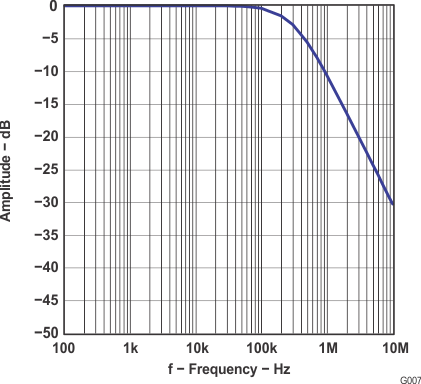Figure 13. Amplitude vs Frequency Antialias Filter Stop-Band Characteristics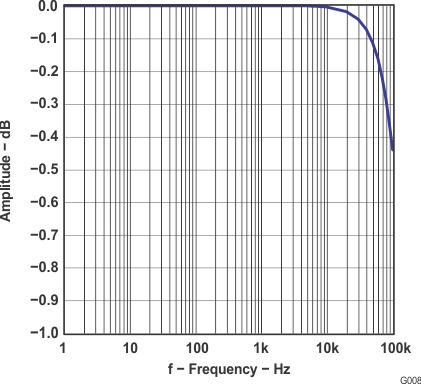Figure 14. Amplitude vs Frequency Antialias Filter Pass-Band Characteristics

#### 6.6.2 Typical Characteristics: Output Spectrum

TA = 25°C, VCC = 5 V, VDD = 3.3 V, Master mode, fS = 44.1 kHz, system clock = 384 fS, oversampling ratio = ×128, and 24-bit data (unless otherwise noted).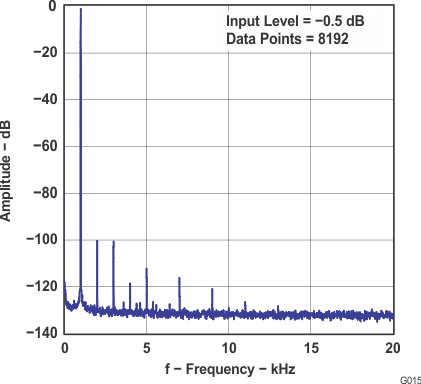Figure 15. Amplitude vs Frequency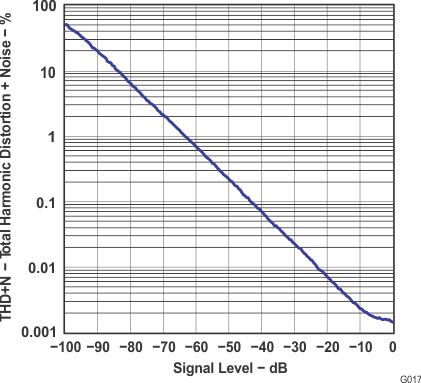Figure 17. Total Harmonic Distortion + Noise vs Signal Level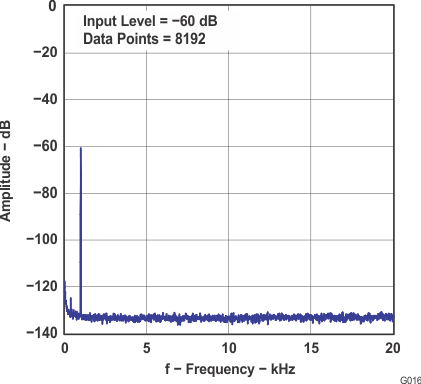Figure 16. Amplitude vs Frequency

#### 6.6.3 Typical Characteristics: Supply Current

TA = 25°C, VCC = 5 V, VDD = 3.3 V, Master mode, fS = 44.1 kHz, system clock = 384 fS, oversampling ratio = ×128, and 24-bit data (unless otherwise noted).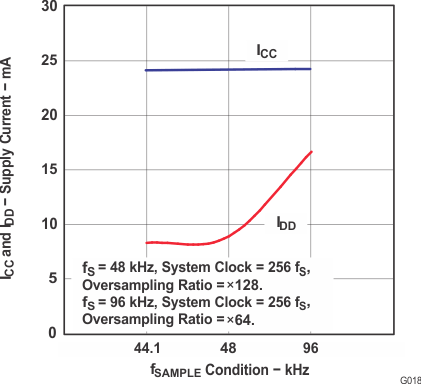Figure 18. Supply Current vs fSAMPLE Condition